# Risk of a statistical procedure

(diff) ← Older revision | Latest revision (diff) | Newer revision → (diff)

A characteristic giving the mean loss of an experimenter in a problem of statistical decision making and thus defining the quality of the statistical procedure under consideration.

Suppose that one has to make a decisionin a measurable decision space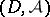with respect to a parameteron the basis of a realization of a random variablewith values in a sampling space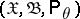,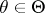. Further, let the loss of a statistician caused by making the decisionwhen the random variablefollows the law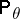be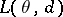, whereis some loss function given on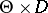. In this case, if the statistician uses a non-randomized decision function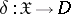in the problem of decision making, then as a characteristic of this functionthe function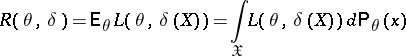is used. It is called the risk function or, simply, the risk, of the statistical procedure based on the decision functionwith respect to the loss.

The concept of risk allows one to introduce a partial order on the set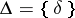of all non-randomized decision functions, since it is assumed that between two different decision functions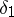andone should preferifuniformly over all.

If the decision functionis randomized, the risk of the statistical procedure is defined by the formula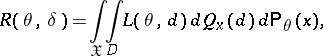where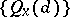is the family of Markov transition probability distributions determining the randomization procedure.

How to Cite This Entry:
Risk of a statistical procedure. Encyclopedia of Mathematics. URL: http://encyclopediaofmath.org/index.php?title=Risk_of_a_statistical_procedure&oldid=12130
This article was adapted from an original article by M.S. Nikulin (originator), which appeared in Encyclopedia of Mathematics - ISBN 1402006098. See original article